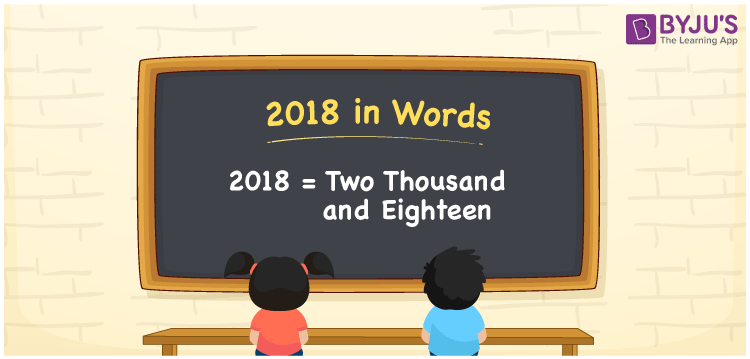# 2018 in Words

2018 in words is written as “Two Thousand and Eighteen”. In Maths, 2018 is a cardinal number that expresses a quantity or a value. For example, the cost of a Showcase is Rs.2018 or Rupees Two Thousand and Eighteen. 2018 can also be used as a counting number like “there are 2018 students sitting in an auditorium”. Learn more about Numbers In Words at BYJU’S.

 2018 in Words Two Thousand and Eighteen Two Thousand and Eighteen in Numbers 2018

## 2018 in English Words## How to Write 2018 in Words?

The number 2018 in words can be written using a place value chart, where we can identify the position of each digit. Since 2018 is a four-digit number, thus the place value of the digits is given by:

 Thousands Hundreds Tens Ones 2 0 1 8

From the above table,

2 → Thousands place

0 → Hundreds place

1 → Tens

8 → Ones place

Hence, when we read the number from right to left, it is Two Thousand and Eighteen.

### Expanded Form of 2018

We can write the expanded form as:

2 x Thousand + 0 × Hundred + 1 × Ten + 8 × One

= 2 x 1000 + 0 × 100 + 1 × 10 + 8 × 1

= 2000 + 0 + 10 + 8

= Two Thousand and Eighteen

2018 is a whole number that is succeeded by 2017 and preceded by 2019. Learn more about the number 2018 below:

• 2018 in Words – Two Thousand and Eighteen
• Is 2018 an odd number? – No
• Is 2018 an even number? – Yes
• Is 2018 a perfect square number? – No
• Is 2018 a perfect cube number? – No
• Is 2018 a prime number? – No
• Is 2018 a composite number? – Yes

## Frequently Asked Questions on 2018 in words

Q1

### What is 2018 in words?

2018 in words is given by Two Thousand and Eighteen.
Q2

### What is the rule to write 2018 in words?

2018 in words is written based on the place values of each digit. 2 is at thousands, 0 at hundreds, 1 at tens and 8 at ones.

2 → Thousands place

0 → Hundreds place

1 → Tens

8 → Ones place

Thus, reading from right to left, it is Two Thousand and Eighteen.

Q3

### What is the value of 2018 + 100 in words?

2018 + 100 = 2118, i.e., Two Thousand One Hundred Eighteen in words